# ISEE Upper Level Quantitative : Data Analysis

## Example Questions

### Example Question #81 : Data Analysis

A data set has twelve elements; the mean and the median of the set are both 50.

A new data set is formed by increasing each element by 5. Which is the greater quantity?

(a) The mean of the new data set

(b) The median of the new data set

(a) is greater

(a) and (b) are equal

(b) is greater

It is impossible to tell from the information given

(a) and (b) are equal

Explanation:

(a) Since each element of the old set is increased by 5, the sum of the elements is increased by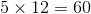. This increases the mean by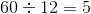, to 55.

(b) The median of the old set is the mean of the sixth- and seventh-highest elements. Since each element of the old set is increased by 5, these elements remain the sixth- and seventh-highest elements; their sum is increased by 10, and their mean is increased by 5, to 55.

The mean and the median of the new set are equal.

### Example Question #81 : Data Analysis

Which is the greater quantity?

(a) The median of the data set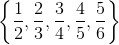(b)(b) is greater

(a) is greater

(a) and (b) are equal

It is impossible to tell from the information given

(a) and (b) are equal

Explanation:

The median of a data set with five elements is its third-greatest element, which here is### Example Question #83 : Data Analysis

Which is the greater quantity?

(a) The median of the data set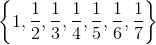(b)(a) and (b) are equal

It is impossible to tell from the information given

(a) is greater

(b) is greater

(a) and (b) are equal

Explanation:

The median of a data set with seven elements is its fourth-greatest element, which here is### Example Question #1 : Median

A data set has nine elements. Four of the elements are greater than 50; four are less than 50.

Which is the greater quantity?

(a) The median of the data set

(b) 50

It is impossible to tell from the information given.

(a) is greater.

(b) is greater.

(a) and (b) are equal.

(a) and (b) are equal.

Explanation:

If four of the elements are greater than 50 and four are less than 50, then the fifth-highest element, which is the median of a nine-element set, must be 50.

### Example Question #84 : Data Analysis

Consider the data set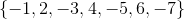Which is the greater quantity?

(a) The median of the data set

(b) The mean of the data set

It is impossible to tell from the information given

(a) is greater

(b) is greater

(a) and (b) are equal

(b) is greater

Explanation:

(a) Arrange the elements in ascending order: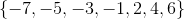The median is the middle element, which is.

(b) Add the elements and divide by 7: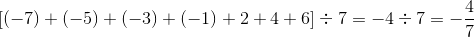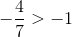, so the mean is greater.

### Example Question #81 : Data Analysis

In the following set of numbers compare the mean and the median of the set: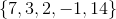It is not possible to tell from the information given

The mean of the set is greater than the median of the set.

The mean of the set is equal to the median of the set.

The median of the set is greater than the mean of the set.

The mean of the set is greater than the median of the set.

Explanation:

The median is the middle value of a set of data containing an odd number of values. In this question in order to find the median we should first put the numbers in order: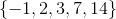So the median is.

Mean of a data set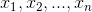is the sum of the data set values divided by the number of data: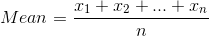So we have: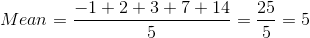So the mean of the set is greater than the median of that.

### Example Question #82 : Data Analysis

In the following set of numbers compare the mean and the median of the set: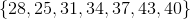It is not possible to tell from the information given

The median of the set is greater than the mean of the set.

The mean of the set is equal to the median of the set.

The mean of the set is greater than the median of the set.

The mean of the set is equal to the median of the set.

Explanation:

The median is the middle value of a set of data containing an odd number of values. In this question in order to find the median we should first put the numbers in order: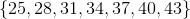So the median is.

Mean of a data setis the sum of the data set values divided by the number of data:So the mean of the set is equal to the median of the set.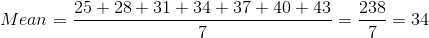So the mean of the set is greater than the median of that.

### Example Question #83 : Data Analysis

Consider the following set of data: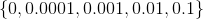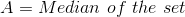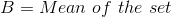CompareandThe mean of the set is greater than the median of the set.

It is not possible to tell from the information given.

The mean of the set is equal to the median of the set.

The median of the set is greater than the mean of the set.

The mean of the set is greater than the median of the set.

Explanation:

The median is the middle value of a set of data containing an odd number of values. In this question in order to find the median we should first put the numbers in order: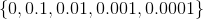So the median is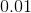.

Mean of a data setis the sum of the data set values divided by the number of data:So the mean of the set is equal to the median of the set.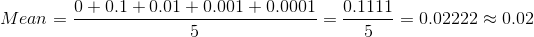So the mean of the set is greater than the median of that.

### Example Question #84 : Data Analysis

In the following set of numbers compare the mean and the median of the set: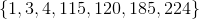The mean of the set is equal to the median of the set.

It is not possible to tell from the information given.

The median of the set is greater than the mean of the set.

The mean of the set is greater than the median of the set.

The median of the set is greater than the mean of the set.

Explanation:

The median is the middle value of a set of data containing an odd number of values which is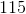in this problem.

Mean of a data setis the sum of the data set values divided by the number of data:So the mean of the set is equal to the median of the set.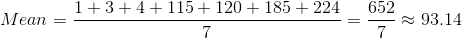So the median of the set is greater than the mean of that.

### Example Question #89 : Data Analysis

In the following set of numbers compare the mean and the median of the set: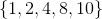The mean of the set is greater than the median of the set.

The median of the set is greater than the mean of the set.

The mean of the set is equal to the median of the set.

It is not possible to tell from the information given

The mean of the set is greater than the median of the set.

Explanation:

The median is the middle value of a set of data containing an odd number of values which isin this set of numbers.

Mean of a data setis the sum of the data set values divided by the number of data:So we have: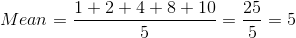So the mean of the set is greater than the median of the set.

### All ISEE Upper Level Quantitative Resources